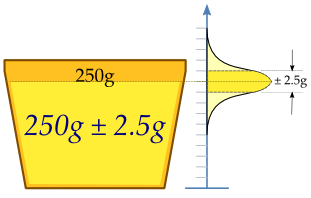I read a lot of health related studies, and most contain in their conclusion a statistical description of the result. To be able to draw valid conclusions for oneself it is very important to understand what all the numbers mean. I have recently learned all that, so here is an example together with an explanation:

The multivariate relative risk of gout among men in the highest quintile of meat intake, as compared with those in the lowest quintile, was 1.41 (95 percent confidence interval, 1.07 to 1.86; P for trend=0.02)

A more understandable translation of the above is this (hover over the links):

For the 20% (quintile: In statistics, a quantile for the case where the sample or population is divided into fifths) who eat the most meat, the probability of getting gout is 1.41 times (41%) higher than the probability for the 20% who eat the least meat. (The number of participants in the study is large enough to say that with 95% probability the populational (real) mean relative risk is between 1.07 and 1.86, with 1.41 being the best estimate within the range. There is a 2% probability (P for trend=0.02) that the whole claim is baloney and all this just happened by chance.)

As posted by jt512: The information in the parentheses is indeed important. 1.41 is the best “point estimate” of the relative risk, given the data. However, the estimate is subject to statistical uncertainty. The 95% confidence interval (CI) quantifies that uncertainty. It is an interval estimate of the relative risk, in the sense that there is a 95% chance that the true relative risk is in the interval.Wide confidence intervals imply that the point estimate is highly imprecise, or uncertain. The given CI, 1.07–1.86, is quite wide. The lower bound is barely above a relative risk of 1, which would mean no effect of meat intake on risk of gout, and the upper bound is close to 2, which would mean that the risk in the highest quintile is double that in the lowest.

Finally, the P for trend shows that the data are weakly statistically significantly consistent with a linear trend in relative risk across quintiles. Statistically significant, because the p-value is less than 0.05; weakly, because it isn’t much lower than 0.05. You should look at the point estimates of the relative risks for each quintile and decide for yourself whether there appears to be a linear trend from quintile to quintile. Lack of a linear trend would tend to undermine a causal role for meat in the development of gout.

Voilá, now go and read some papers!

Sources: